# Orthogonal

## Geometry and Motion

Orthogonal

### Lorentzian and Riemannian Geometry

In the universe we inhabit, the names we use for directions in space — up, down, left, right, forwards, backwards, north, south — are all defined in terms of particular objects, such as our own bodies, or the planet we’re standing on. We do this because it’s convenient, but ultimately we have no other choice: we need to get our bearings from objects, because the underlying geometry of space itself treats all directions equally.

Less obviously, when we talk about “the future” or “the past” we’re implicitly referring to the future or past of a particular object, or group of objects. When we talk about moving, say, one hour into “the future”, we usually mean staying fixed in space compared to the streets and buildings around us. But the direction in space-time traced out by a speeding car is just as good a choice for “the future” as that traced out by a lamp-post on the side of the road — and again, the underlying geometry of space-time treats all such directions equally.But while you might say “left” for what I call “forwards”, and you might think you’re “motionless, moving only into the future” when I think you’re zipping past me in interstellar space at a thousand kilometres per second, it’s not true that any direction in space-time is indistinguishable from any other. The bottom line is that the two of us will always agree on the distinction between timelike, spacelike and null directions.

Timelike directions are those traced out over time by the ordinary objects around us. We might say that a car is “heading east”, but of course we know it’s heading into the future as well, so on a space-time diagram it will trace out a line that slants a little eastwards as it moves into the future.

The spacelike direction “east” is something we can draw on a diagram, but no real object can follow a path through space-time that advances only eastwards without advancing through time.

In between the timelike and spacelike directions are those traced out by light; these are known as lightlike or null directions.

Suppose someone using a system of units where the speed of light is equal to one (such as years for time and light-years for distance) measures two events in space-time as being separated by a distance x and a time t. Then we can say of the vector pointing between the two events:

• if x2t2 is negative, the vector is timelike (such as the vectors from A to B and A to C in the diagram);
• if x2t2 is positive, the vector is spacelike (such as the vector from A to E in the diagram);
• if x2t2 equals zero, the vector is null (such as the vector from A to D in the diagram).

At the heart of special relativity is the discovery that everyone moving with a uniform velocity will measure the same value for x2t2, and hence they will agree on this classification of vectors as timelike, spacelike or null. The kind of space-time geometry where x2t2 is an invariant — a quantity all observers can agree on — is known as Lorentzian geometry.In contrast to this, in a universe with Riemannian geometry the quantity that everyone agrees on is x2+t2. This is just the square of the distance we’d measure in Euclidean space between the two points, by way of Pythagoras’s Theorem.

(If the geometry is curved, this will only apply if we measure x and t on a small enough scale, just as the curved surface of the Earth will only obey Euclidean geometry if we limit ourselves to a small enough region.)

This squared distance, x2+t2, is always positive (excluding the trivial case when both x and t are zero). So the old distinctions between timelike, spacelike and null directions are gone; in the Riemannian universe, all directions are essentially the same. There is no fundamental difference between a direction that someone might call “east” and one that someone might call “the future”.

In the Lorentzian case, we made use of the speed of light to determine the units in which x and t should be measured. In the Riemannian case, there is no single, universal speed of light. So how do we choose the proper units in which to measure distances and times? We simply adjust the ratio between our units for time and our units for distance until we find, experimentally, that Pythagoras’s Theorem always holds — even when it involves time as well as space. Throughout these notes, all the equations we give for Riemannian physics will assume that such a choice of units has been made.

So far, we’ve been simplifying things a little by assuming that the vectors we’re concerned with span only two directions in our coordinate system. Also, the vectors we’ve discussed so far have all been displacement vectors, which are like arrows we can imagine going from event A to event B. In these notes we’ll be talking about all kinds of vectors, whose components are associated with the four dimensions but which might measure something other than distance. So in general, we’ll describe the components of a vector v as vx, vy, vz and vt. (Note that these superscripts are just labels for the four components, and have nothing to do with raising anything to a power.) The squared length of the four-dimensional vector v is then given by the generalisation of Pythagoras’s Theorem from two dimensions to four:

|v|2 = (vx)2 + (vy)2 + (vz)2 + (vt)2

Everyone will agree on the length of a vector, |v|, even when they use different coordinate systems and measure different values for the individual components vx, vy, vz and vt.

### Dot Products

In Riemannian geometry, as well as agreeing on the lengths of vectors, people using different coordinate systems will also agree on the angles between vectors. This allows us to define an invariant quantity that is related to both lengths and angles.

If we have two vectors, v and w, we define the dot product of v and w as follows: for each of the four dimensions x, y, z and t we multiply the components of the two vectors together, then we add them all up. We write this as v · w, so:

v · w = vx wx + vy wy + vz wz + vt wt      (Riemannian dot product)

Using this definition, the squared length of a vector is just its dot product with itself:

|v|2 = v · v

The dot product is left unchanged by rotations. To see this, consider a triangle, two of whose sides are the vectors v and w; the third side will be vw (as in the diagram below right). The squared length of the third side is:

|vw|2 = (vw) · (vw) = |v|2 + |w|2 – 2 v · w

Hence v · w = (|v|2 + |w|2 – |vw|2) / 2, and since none of these lengths change when the triangle is rotated, the dot product can’t change either.If the angle between v and w is θ, then:

v · w = |v| |w| cos θ

This isn’t an obvious claim, but it’s easy to prove. Suppose we consider the simplest possible case, where w lies on the positive half of the x-axis (so wy=wz=wt=0 and wx>0), and v lies in the xt-plane (so vy=vz=0). This is illustrated in the diagram on the right.

Because of all the zero components, we have:

v · w = vx wx
|w| = wx

From the definition of the cosine of an angle, we also have:

vx = |v| cos θ

from which it follows that, in this particular case:

v · w = |v| |w| cos θ

But given any v and w whatsoever, we could always rotate them to make them conform to the restrictions we’ve assumed above. That won’t change the lengths of the vectors or the angle θ between them, and nor will it change their dot product, so if the result holds true after that rotation it must have been true all along.

The cosine of an angle is positive when the angle is less than 90°, zero when the angle is exactly 90°, and negative when the angle lies between 90° and 180°. So:

• if v · w > 0 then v and w are pointing in roughly the same direction (the angle between them is less than 90°);
• if v · w = 0 then v and w are perpendicular;
• if v · w < 0 then v and w are pointing in roughly opposite directions (the angle between them is more than 90°).Suppose the vector u is a unit vector, a vector of length 1. Any other vector v can always be broken down as the sum of two vectors, one parallel to u and one perpendicular to u. The part of v parallel to u is known as the projection of v onto u. We can explicitly write the two parts of v as follows:

v = (u · v) u + (v – (u · v) u)

The first part is clearly parallel to u because it’s just a number, u · v, multiplied by u. The dot product u · v is almost the length of the projection of v onto u; the only difference is that u · v will be negative if the two vectors point in roughly opposite directions, so in that case it is the opposite of the projection’s length.

The second part can be seen to be perpendicular to u by taking its dot product with u.

u · (v – (u · v) u)
= (u · v) – (u · v) (u · u)
= (u · v) – (u · v)
= 0

We will call the unit vectors that point along the four axes of our coordinate system ex, ey, ez and et; these four vectors are known as the basis vectors of the coordinate system. Then for any vector v, its components in this coordinate system are given by its dot products with the basis vectors:

vi = ei · v, where i=x, y, z, t

In the Riemannian universe, all of this vector geometry is very simple: it’s just the Euclidean geometry of space that we’re accustomed to, with one more dimension added for time. In contrast to this, in the Lorentzian universe we always have to take care with the sign differences in the time dimension. For example, in the Lorentzian universe the dot product of two four-dimensional vectors (or “four-vectors”) has a negative sign for the time coordinates:

v · w = vx wx + vy wy + vz wzvt wt      (Lorentzian dot product)

### Time Without Timelike Directions

The idea of a universe without any notion of time built into its geometry might conjure up an image of something resembling a snapshot taken in our own universe: a single moment, frozen and unchanging. But on reflection, that makes no sense at all. When seen in all four dimensions, our own universe is also unchanging; what constitutes change for us is the fact that successive three-dimensional slices through the four-dimensional universe are different. So if the laws of physics that govern the contents of the Riemannian universe also allow successive three-dimensional slices to differ, then the same possibility will exist for something that is perceived as change.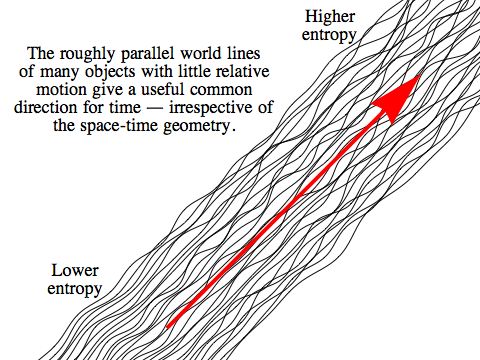Relativity tells us that there’s no single “right way” to slice up our own universe into successive moments of time. Different people in relative motion will generally do this in different ways, with everyone treating their world line — the path, or history, they trace out through space-time — as marking the time direction, and all the directions perpendicular to that as being directions in space.

Now it might not be immediately obvious what kind of objects a Riemannian universe will contain, but it turns out that it will also be full of world lines. (The detailed reasons for this lie in quantum mechanics, but we’ll get a glimpse of the way world lines emerge when we look at waves, on the next page.) The main difference is that while world lines in the Lorentzian universe must run in timelike directions, in the Riemannian universe there is no restriction on the directions they can follow.

Of course we normally think of time as universal, rather than a property of our own world line, because in everyday life all the people and objects around us have very small velocities relative to each other, so all our world lines are running along in a tight bundle, weaving back and forth a tiny bit but basically parallel to each other. That is what gives us, for most practical purposes, a single, common idea of time. It’s that shared motion that’s most important to ordinary experience, not the fact that the underlying space-time geometry classifies a certain (much larger!) set of directions as timelike. And a similar bundle of nearly parallel world lines in the Riemannian universe would give rise to the same practical sense in which the direction of the bundle constituted a useful shared notion of time.

So, let’s take it for granted that we can imagine ourselves to be inhabitants of the Riemannian universe, with world lines of our own. Just as we do in our own universe, we would normally use a time coordinate whose axis points in the direction of our world line, and space coordinates whose axes are orthogonal to it. We’ll also assume that we have some clear-cut way of choosing one direction along our world line as “the future”. As in our own universe, that will come down to a question of entropy: the future will be the direction in which entropy is increasing.

Any object whose world line is parallel to our own, we would consider to be stationary, since in our coordinates its position in space won’t be changing over time. Any object whose world line is inclined at some angle to our own, we would consider to be moving.We have our own time coordinate, t, but relativity dictates that the passage of time for any object will be measured along its world line. We call the time measured this way the proper time, τ. In the Riemannian universe, the proper time that passes for an object between two events is just the length of the world line for the object in question — and apart from the issue of choosing the right units, this is the same as the length measured along any other curve.

Now, consider a unit vector u tangent to the object’s world line. We call this the object’s four-velocity, because it’s a four-dimensional vector that describes the object’s instantaneous state of motion. We can extract the ordinary (three-dimensional) velocity v of the object in a particular coordinate system by dividing the spatial components of u by the time component; in the diagram, where everything is confined to the xt-plane, we would have:

vx = ux / ut

because if the object continued along the tangent line marked out by u, it would travel a distance ux in a time ut.

Of course the object in the diagram isn’t maintaining a constant velocity, it’s accelerating. The four-acceleration of an object, which we’ll call a, is defined as the rate at which the object’s four-velocity is changing with respect to proper time, τ. We write this as:

a = ∂τu

Don’t be alarmed by the notation here! The symbol “∂” is just a concise way of writing “rate of change”, while the subscript, τ, means we’re talking about the change that occurs in u when we vary τ.

Since the four-velocity u is always a unit vector, the only change it can experience is in direction, rather than length, which means the four-acceleration a must be orthogonal to u.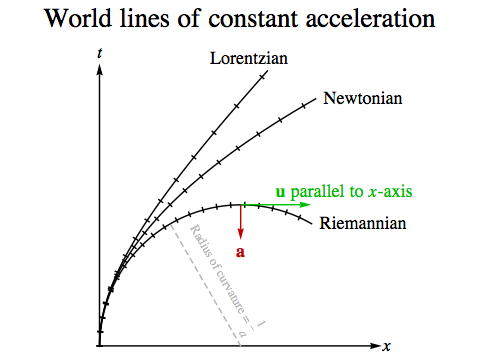Suppose we take an object that is initially at rest in our coordinate system, and subject it to constant acceleration in our x direction. To be precise, we require the magnitude of the object’s acceleration to be a constant, a, and the acceleration four-vector to remain in the xt-plane. The object’s world line will start out parallel to our t-axis, and then gradually tilt towards the x-axis.

In the Lorentzian universe, the object will move ever faster, but it will never reach the speed of light.

In a Newtonian universe, there is no upper limit on the object’s speed, but at any finite time its speed will still be finite.

In the Riemannian universe, the object’s world line will be a circular arc. This shouldn’t be surprising, because the geometry and mathematics in play here is very familiar — it’s more or less the same as the mathematics of centrifugal force, when a constant sideways tug on a moving object will cause it to follow a circular path.

We can write the object’s (x, t) coordinates, four-velocity and four-acceleration explicitly as functions of proper time:

x(τ) = 1/a (1 – cos a τ, sin a τ)
u(τ) = ∂τx(τ) = (sin a τ, cos a τ)
a(τ) = ∂τu(τ) = a (cos a τ, –sin a τ)

So after a finite time — 1/a according to us, or π/(2a) according to the object itself — the object will end up with a four-velocity parallel to our x-axis, and we will consider it to have an infinite velocity. After that, it will end up moving backwards in time in our coordinates!

Now, under what circumstances constant acceleration like this can be maintained in practice in the Riemannian universe will depend on the mechanism that’s meant to be providing the force on the object, and as in our universe any such process will be subject to stringent laws regarding energy and entropy. But as a statement of pure kinematics, the diagram shows what happens if constant acceleration can be achieved.

### Energy-Momentum Vectors

Suppose we associate with every object around us an arrow whose length is equal to the object’s mass, m, and whose direction is tangent to the object’s world line. We will always choose these arrows to point in rough agreement with our personal choice of “the future”; to be precise, we will always choose their direction so they don’t make an angle greater than 90° with our time axis.This arrow is the object’s energy-momentum vector, and we’ll give it the symbol P. We have already defined a unit vector tangent to the object’s world line, the four-velocity u, so we can write:

P = m u

To keep things simple, let’s assume for the moment that the object’s world line lies in our xt plane, so we only have to deal with two dimensions. We will call the t component of the energy-momentum vector the object’s total energy, E, and the x component of the energy-momentum vector the object’s momentum, p. Then:

Pt = E
Px = p
m2 = |P|2 = (Pt)2 + (Px)2 = E2 + p2

Now, the object’s velocity v will be the ratio ux / ut between the x and t components of its four-velocity. Because the energy-momentum vector is a multiple of the four-velocity, we can get this ratio straight from that energy-momentum vector’s components:

v = p / E

Making use of our last two equations, we have:

p = Ev
E2 = m2p2 = m2E2v2
E2 (1 + v2) = m2

giving us E and p in terms of m and v:

E = m / √(1 + v2)
p = mv / √(1 + v2)

You might recognise these formulas as a simple variation on the usual relativistic versions, with 1 + v2 in place of 1 – v2.

Using a Taylor series for 1 / √(1 + v2), we can approximate these formulas for small values of v:

1 / √(1 + v2) ≈ 1 – (1/2) v2
Em – (1/2) mv2
pmv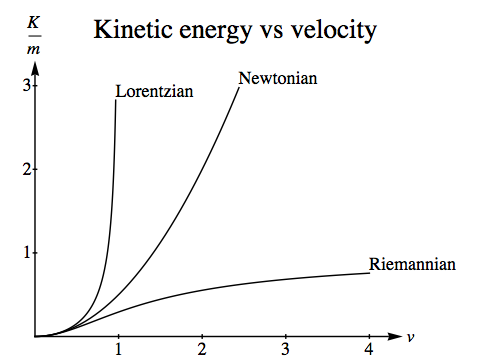The approximate value for the momentum here, mv, is just the momentum of an object in ordinary Newtonian physics. And in Newtonian physics, the kinetic energy of an object is:

KNewtonian = (1/2) mv2

so the approximate value for the total energy is:

EmKNewtonian

In our own universe, we think of an object as having a rest-mass energy mc2 (= m in units where c=1), to which we add the object’s kinetic energy to find its total energy. In the Riemannian universe, we have to subtract the kinetic energy from the rest-mass energy. We define the exact, Riemannian kinetic energy K as:

K = mE = m (1 – 1 / √(1 + v2))

In both Newtonian physics and Lorentzian physics, as the velocity of an object increases its kinetic energy can grow without bound. In Newtonian physics, there is no limit to the velocity, and so KNewtonian can be made as large as you wish. In Lorentzian physics v<1, but as it approaches the speed of light (which is 1, in appropriate units) the kinetic energy:

KLorentzian = m (1 / √(1 – v2) – 1)

can again be made as large as you wish. In Riemannian physics, as in Newtonian physics, there is no limit to the velocity you can measure for an object; if its world line is perpendicular to your own, its velocity will be infinite. But however large its velocity, its kinetic energy will never be greater than its rest mass, m.

What about potential energy? We can define potential energy in the usual way, in terms of any forces acting on the particles we’re interested in, and then the sum of kinetic and potential energy will be conserved. Later, we’ll delve into the Riemannian equivalent of electromagnetism, and see the form that a kind of electrostatic potential energy will take. But we can immediately note one curious fact. A system of two particles bound together will generally have less potential energy than the same system where the particles are free, because in the bound state the particles are “stuck in an energy well” with walls sloping down around them. In Lorentzian physics that leads to a “mass defect”: the bound system’s mass is less than the sum of its parts. In Riemannian physics, because kinetic and potential energy have the opposite sense to rest-mass energy, the mass defect will work the other way: a bound system’s mass will be more than the sum of its parts.

For small velocities Riemannian physics will look just like Newtonian physics, and the fact that the kinetic energy is “upside down” need not be apparent. When a fixed number of particles are just bouncing off each other, kinetic energy is simply passed from one particle to another, or converted into potential energy. But in any context where new particles can be created, kinetic energy’s relationship to rest-mass energy can have striking consequences: the creation of the new particle will be balanced, not by a reduction in the kinetic or potential energy of the system creating it, but by an increase. We’ve already noted that in Riemannian physics a bound system has more mass than its individual parts, so matter in any kind of bound state that’s capable of, say, emitting light, will be at risk of turning its extra mass into light and breaking itself apart in the process! The consequences of this – and the mystery of how matter can be stable at all under these conditions – are explored in much more detail in the novel.

### Conservation Laws

Having defined the energy-momentum vector, we should be able to state what it means for energy and momentum to be conserved by some interaction between objects in the Riemannian universe. We could insist on picking a particular reference frame in which we assign energy-momentum vectors to all the objects of interest — making sure that they point into the future of this reference frame — and then we would say that the conservation laws are satisfied if the sum of all the energy-momentum vectors for the various objects before the interaction equals the sum after the interaction.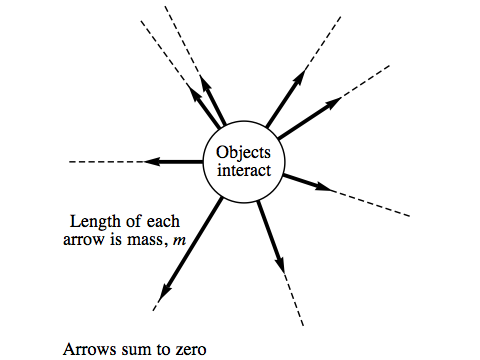There is, however, a much more elegant way to do this, without invoking any particular reference frame at all. We simply draw an arrow along each object’s world line, make its length equal to the object’s mass, and choose all the arrows to point away from the interaction. If energy and momentum are conserved, the sum of all these arrows will be zero.

Having established this symmetrical formulation of the conservation law, we can then see what it implies for any particular observer who does come along and assign energy-momentum vectors “before” and “after” the interaction.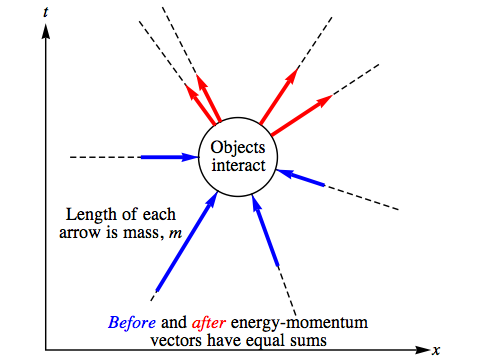The original arrows all point away from the interaction, so any that the observer considers to be associated with objects before the interaction will need to be reversed, to make them point into the observer’s future. But since the sum of all the original arrows is zero, the sum of these reversed arrows will be equal to the sum of all the rest. So to the observer, whatever direction they call “the future”, energy and momentum will be the same before and after the interaction.

What does an observer do with a world line that lies exactly perpendicular to their chosen time axis? All that can really be done in this situation is to arbitarily decide either to treat all such objects as emerging from the interaction, in which case their arrows are left unchanged and are taken as energy-momentum after the interaction – or to treat them all as arriving at the interaction, so their arrows are reversed, and then taken as energy-momentum that was present before the interaction. Either way, energy and momentum will be seen to be conserved.

### Strange Collisions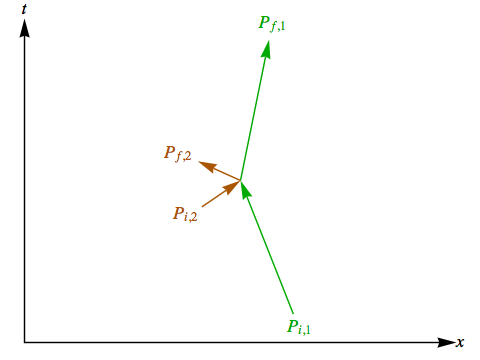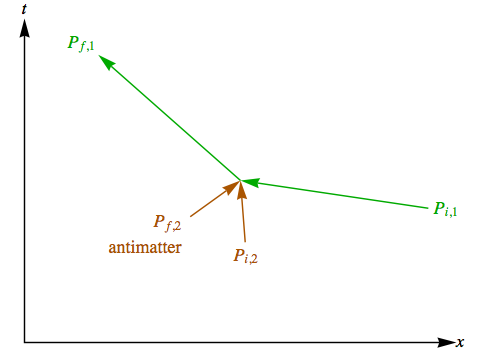Suppose there is a collision in the Riemannian universe between two point-like objects that collide head-on. Let’s assume a perfectly elastic collision, where no energy is converted to other forms, neither object breaks apart, and the two don’t end up stuck together.

Under those assumptions, if we know one energy-momentum vector for each of the particles involved in the collision, by using the conservation laws we can find the other vectors. In the diagram on the upper right, for example, if we know the initial energy-momentum vectors Pi, 1 for the first particle and Pi, 2 for the second particle, we can calculate what their final energy-momentum vectors Pf, 1 and Pf, 2 must be. In the collision shown in this diagram, energy and momentum are conserved, and so anyone in the Riemannian universe who arranges for two particles to collide in this fashion would expect the outcome to be pretty much as it’s portrayed here.

In the diagram on the lower right, we’ve redrawn exactly the same collision as before, except that it’s been rotated by about 60 degrees and we’ve had to swap the direction on the Pf, 2 arrow to give it a positive energy in the new reference frame. It’s still a perfectly valid collision, obeying the conservation laws ... but for an observer using the time axis shown here, there are now three world lines coming together and only one remaining after the collision. In this view, the second object has collided with an antimatter version of itself and the two have annihilated, with the resulting energy being absorbed by the first object, greatly decreasing its speed (total energy having the opposite sense to kinetic energy).

For two observers to have different accounts of the same events is common in relativity, but the real twist comes when we imagine what it means for someone trying to arrange a collision like this. In the first view, we can say that anyone who sets up an experiment with the initial energy-momentum vectors as shown can use the conservation laws to predict the outcome. But in the second view, the very same diagram is telling us that anyone who succeeds in arranging for two particles to collide with the energy-momentum vectors Pi, 1 and Pi, 2 will only do so if that success is accompanied by the arrival, before the collision, of an antimatter version of the second particle to take part in the collision as well. No one will ever collide the first two particles together, at these particular speeds, unless the third ingredient is also present.

Our intuition, based on our experience of our own universe, might protest that it really ought to be possible for someone to arrange an experiment in which only two of the three particles are present at the collision. But the conservation laws say that this is physically impossible. In our universe, we’re used to the laws of physics constraining what we can do, but not in this way!

There is extra material on this topic for readers who don’t mind a slightly higher level of mathematics.Orthogonal / Geometry and Motion / created Wednesday, 6 April 2011